# Cluster of Six

R
clustering
correlation
Exploring parliamentary voting patterns with hierarchical clustering
Author

Carl Goodwin

Published

January 29, 2018

Modified

February 10, 2023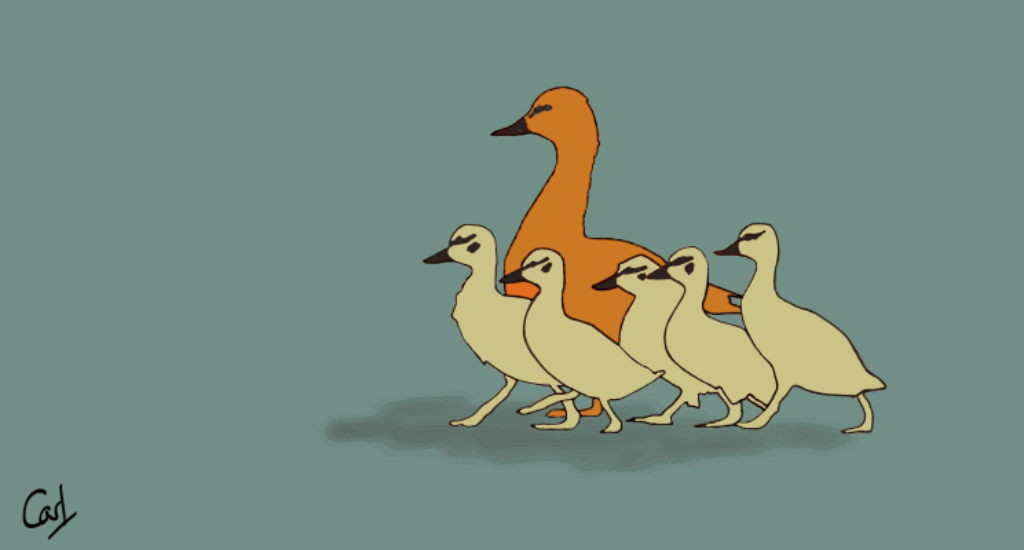Before each vote, the Speaker of the House yells “Division! Clear the Lobby”. I’d like to find which cluster of MPs (Members of Parliament) may be exiting the lobby and going their own way.

Hansard reports what’s said in the UK Parliament, sets out details of divisions, and records decisions taken during a sitting. The R package hansard package provides access to the data.

``````library(conflicted)
library(tidyverse)
conflict_prefer_all("dplyr", quiet = TRUE)
library(clock)
library(wesanderson)
library(hansard)
library(dendextend)
library(corrplot)
library(broom)
library(factoextra)
library(glue)
library(ggrepel)
library(usedthese)

conflict_scout()``````
``````theme_set(theme_bw())

(cols <- wes_palette(name = "Moonrise2"))``````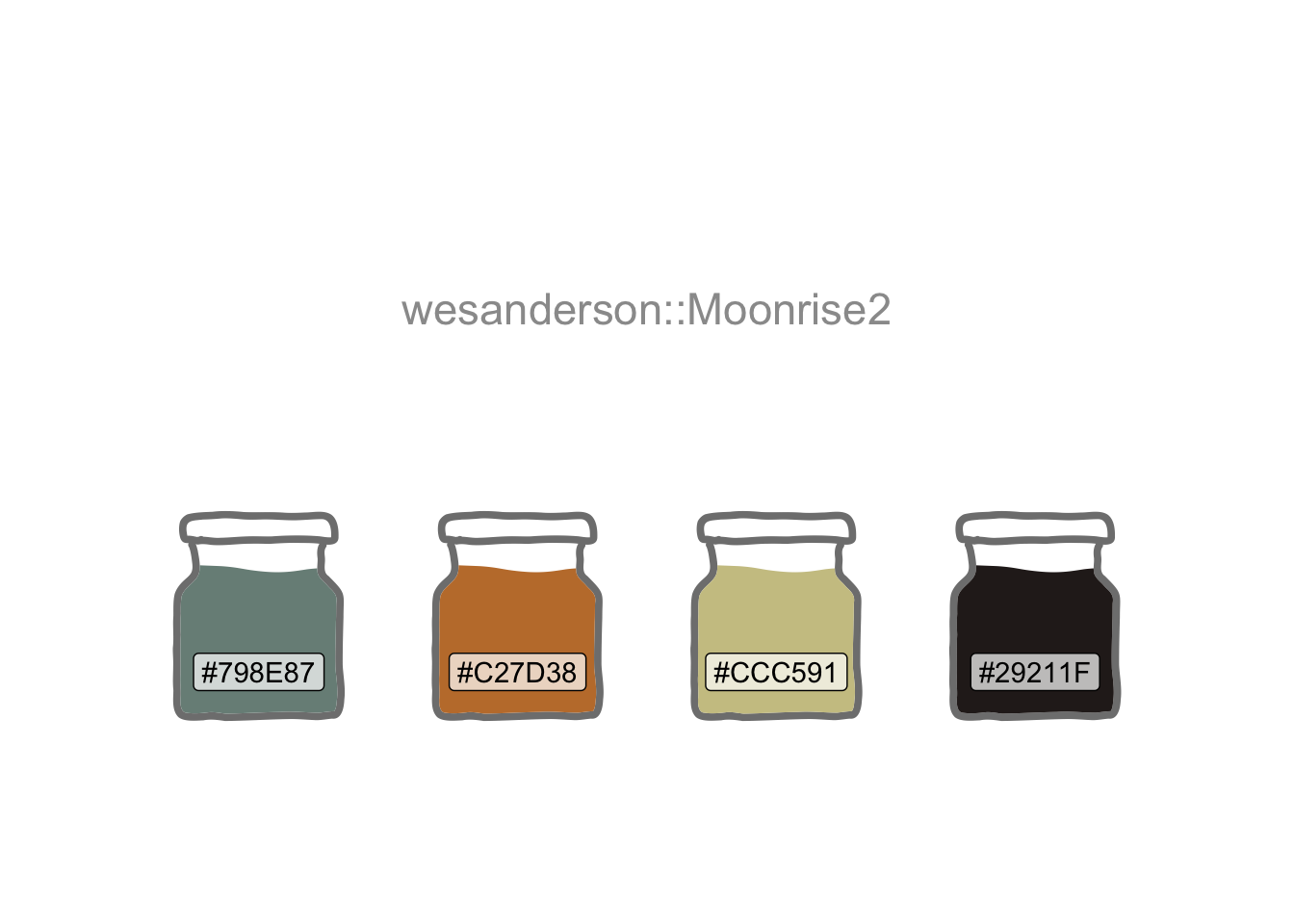I’ll start by building a list of all Labour Party MPs.

``````url_prefix <- "http://data.parliament.uk/members/"

mps <- commons_members() |>
filter(party_value == "Labour" | about == str_c(url_prefix, "478")) |>

saveRDS(mps, file = "mps.rds")``````

Creating a function will enable me to iterate through the MP list to extract their voting records.

``````start_date <- "2017-06-08"
end_date <- "2018-01-28"

mp_vote_record(x,
start_date = start_date,
end_date = end_date,
verbose = FALSE
) |>
mutate(mp = x)
}``````

I’ll use it to extract the “aye” and “no” votes. Use of `possibly` prevents the code from stopping when it encounters former MPs for whom no data is returned.

``````votes <-
compact() |>
map(simplify, "tibbles") |>
list_rbind() |>
rename("lobby" = "vote")

Voting the opposite way to the majority of the party, as well as non-votes, will both be of interest when assessing which MPs are “most distant” from the wider party.

``````votes_df <- votes |>
left_join(mps, by = join_by(mp == ID)) |>
lobby, mp, name = full_name_value) |>
transmute(
vote = if_else(lobby == "aye", 1, -1),
mp = str_c(name, " (", mp, ")"),
title = str_c(title, " (", about, ")")
) |>
pivot_wider(names_from = title, values_from = vote, values_fill = 0)``````

The data are standardised (i.e. scaled) to ensure comparability. This is verified by ensuring the mean and standard deviation are close to zero and one respectively.

``````scaled_df <-
mutate(across(-mp, scale))

scaled_df |>
summarise(across(-mp, list(mean = mean, sd = sd))) |>
summarise(
sd_min = min(pick(ends_with("_sd"))),
sd_max = max(pick(ends_with("_sd"))),
mean_min = min(pick(ends_with("_mean"))) |> round(1),
mean_max = max(pick(ends_with("_mean"))) |> round(1)
)``````
sd_min sd_max mean_min mean_max
1 1 0 0

I’d like to assess whether the data contain meaningful clusters rather than random noise. This is achieved quantitatively by calculating the Hopkins statistic, and visually by inspection.

If the Hopkins statistic is closer to 1 than 0, then we have data which may be clustered.

``````scaled_df |>
select(-mp) |>
pluck("hopkins_stat")``````
`` 0.783357``

A visual assessment of clustering tendency reveals distance data exhibiting a visible structure.

``````scaled_df |>
select(-mp) |>
dist() |>
fviz_dist(
show_labels = FALSE,
low = cols,
mid = cols,
high = cols
)
)``````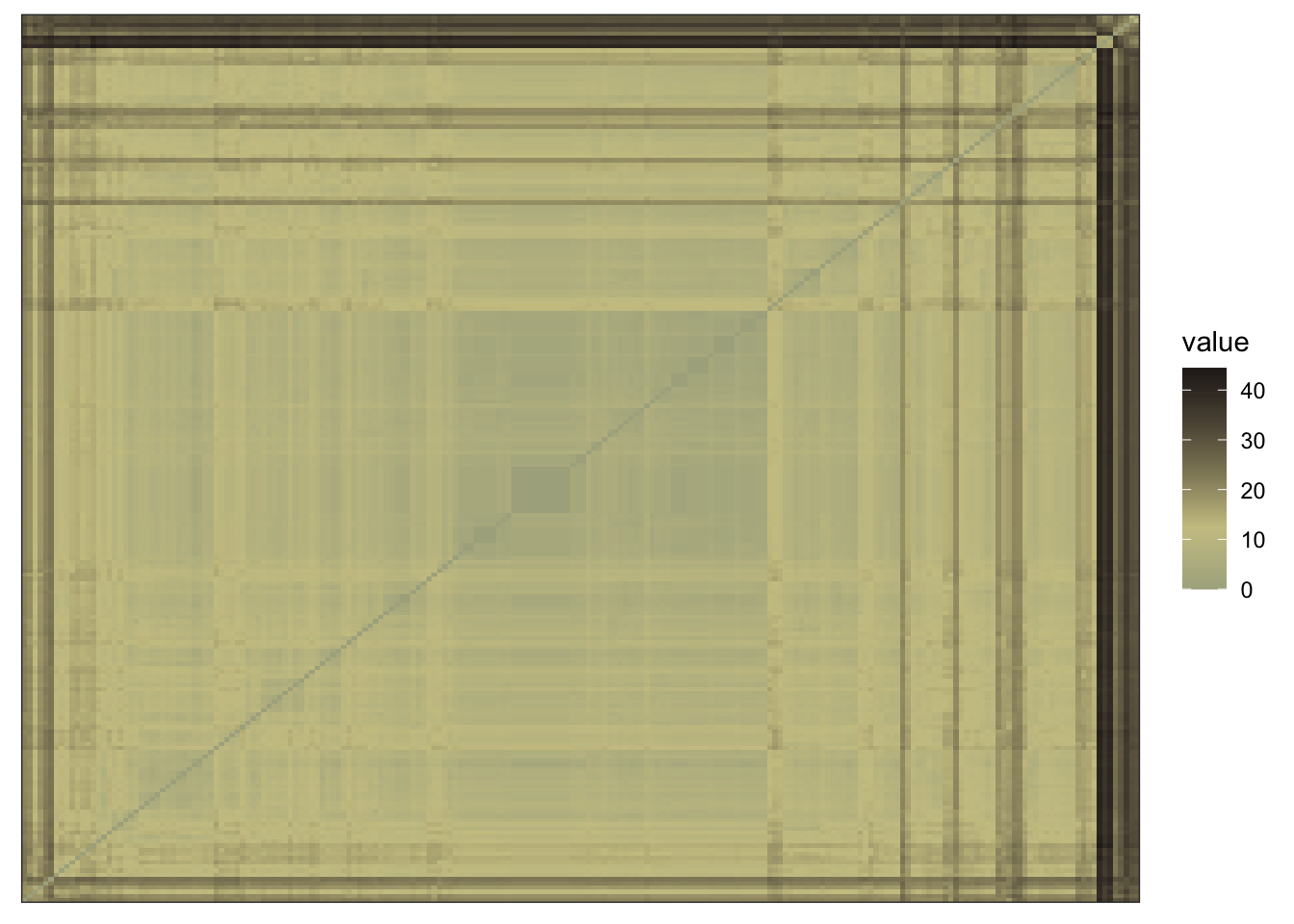There are eight methods I could use for hierarchical clustering, and I’ll need to determine which will yield results that best fit the data.

The correlation plot below shows that the median and ward methods have a weaker correlation with the other five methods.

``````orig_dist <- scaled_df |>
select(-mp) |>
dist()

dend_meths <-
c(
"complete",
"average",
"single",
"ward.D",
"ward.D2",
"mcquitty",
"median",
"centroid"
)

dend_list <-
map(dend_meths, \(x) {
orig_dist |>
hclust(x) |>
as.dendrogram()
})

dend_list |>
reduce(dendlist) |>
set_names(dend_meths) |>
cor.dendlist() |>
corrplot(
"pie",
"lower",
col = cols,
mar = c(1, 0.5, 4, 0.5),
order = "AOE",
tl.cex = 0.8,
tl.col = "black",
cl.cex = 0.7
)``````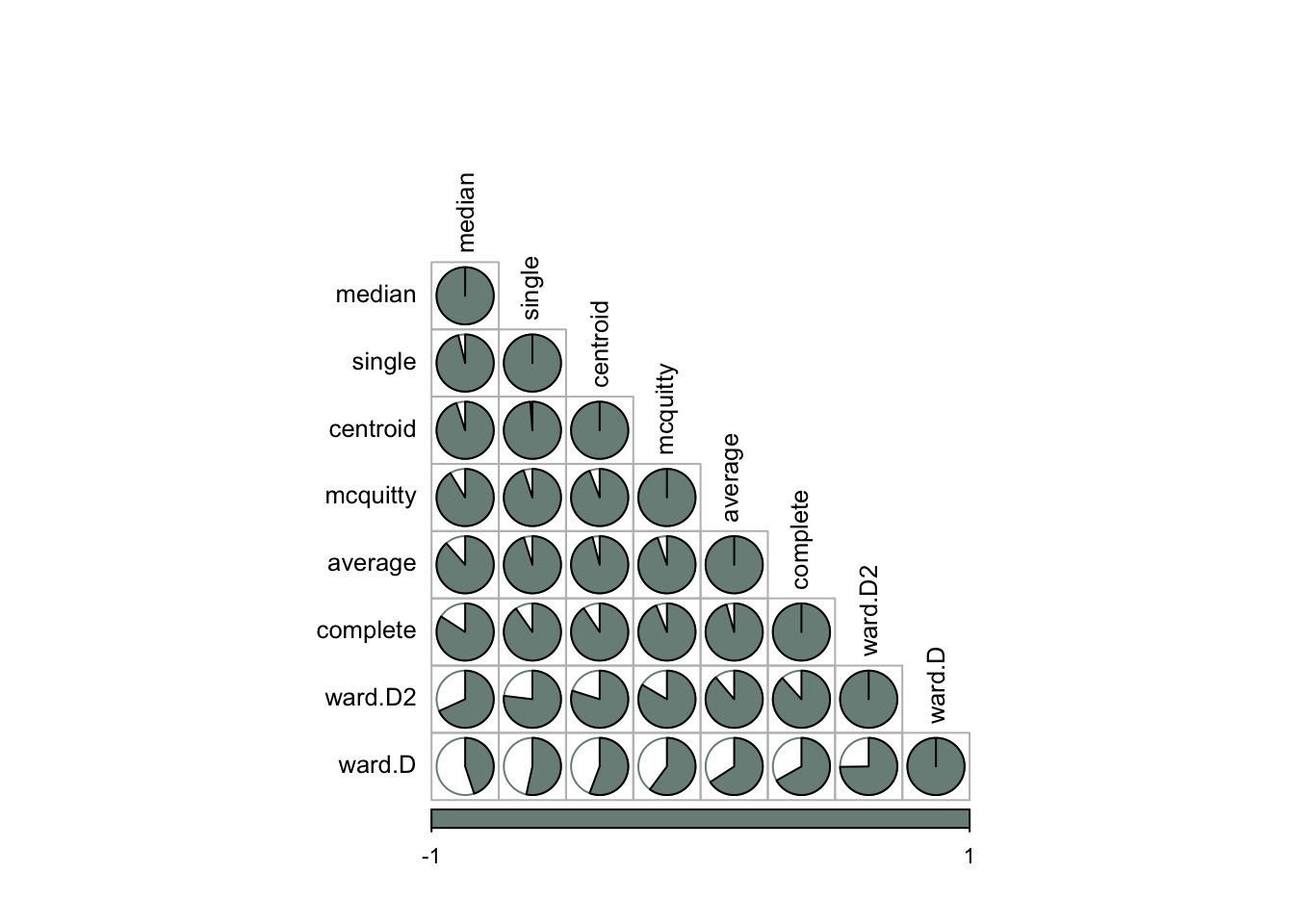The above plot does not tell us which method is optimal. For that, I’ll take each of the cluster agglomeration methods and calculate their cophenetic distances. I can then correlate these with the original distance to see which offers the best fit.

``````methods <- list(
"complete",
"average",
"single",
"ward.D",
"ward.D2",
"mcquitty",
"median",
"centroid"
)

best_method <- map(methods, \(x) {
co_comp <-
orig_dist |>
hclust(x) |>
cophenetic()
tibble(
correlation = cor(orig_dist, co_comp),
method = x
)
}) |>
list_rbind()``````

The plot below confirms the ward and median methods having a weaker fit. Average produces the strongest correlation coefficient of 0.98.

``````best_method |>
ggplot(aes(reorder(method, correlation), correlation)) +
geom_col(fill = cols, width = 0.8) +
geom_text(aes(label = str_c(method, "  ", round(correlation, 2))),
hjust = 1.3, colour = "white"
) +
coord_flip() +
labs(
x = "Method", y = "Correlation",
title = "Cluster Method Correlation Coefficients",
caption = "Source: Hansard"
)``````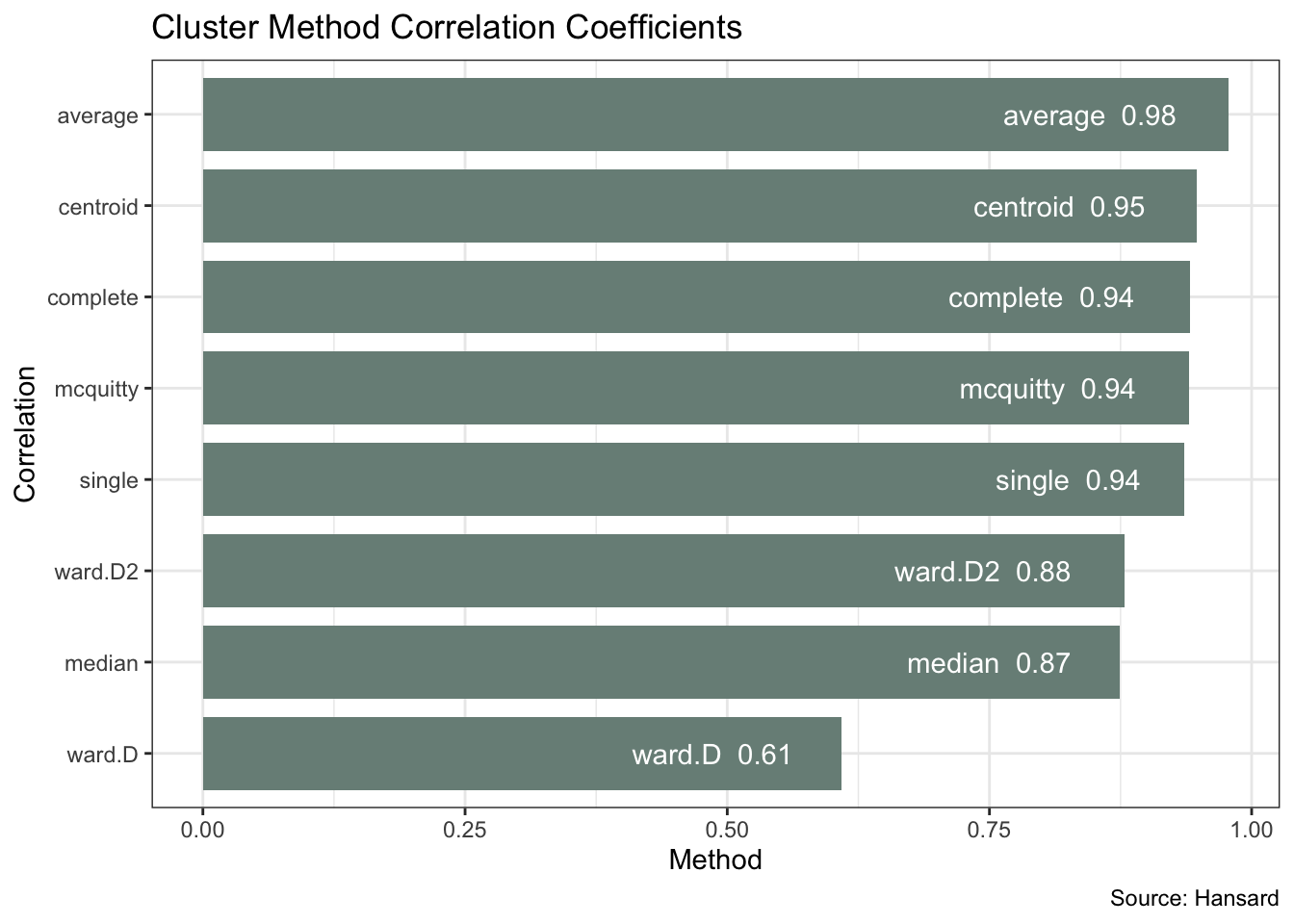I can now plot the full Labour Party dendrogram using the average method. This shows a “cluster of six” MPs which is the last to merge with the rest of the party based on their voting pattern.

``````dend_avg <- orig_dist |>
hclust("average") |>
as.dendrogram()

labels(dend_avg) <- scaled_df\$mp[order.dendrogram(dend_avg)]

dend <- dend_avg |>
color_branches(k = 2, col = cols) |>
set("labels_cex", 0.4)

start_formatted <- date_parse(start_date, format = "%Y-%m-%d") |>
date_format(format = "%b %d, %Y")

end_formatted <- date_parse(end_date, format = "%Y-%m-%d") |>
date_format(format = "%b %d, %Y")

ggplot(rev(dend), horiz = TRUE, offset_labels = -0.2) +
labs(
y = "\nDistance", title = "Hierarchical Clustering of Labour MPs",
subtitle = "Based on House of Commons Divisions Since the 2017 Election",
caption = glue(
"Source: Hansard ({start_formatted} to {end_formatted})")
) +
theme(panel.border = element_blank())``````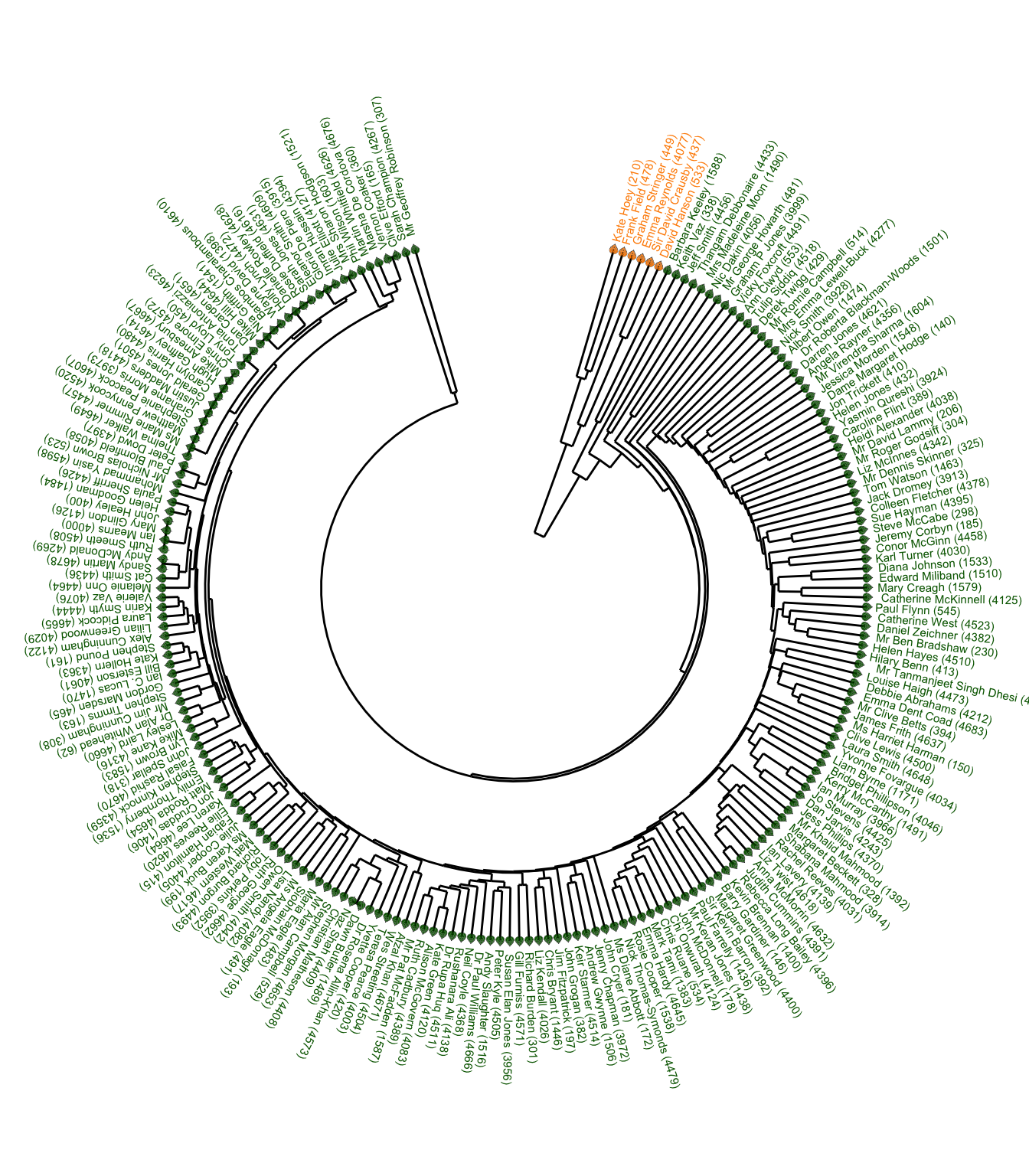I’ll zoom in on the “cluster of six”.

``````dend_cuts <- dend |>
assign_values_to_leaves_nodePar(19, "pch") |>
assign_values_to_leaves_nodePar(5, "cex") |>
assign_values_to_leaves_nodePar(cols, "col") |>
set("labels_cex", 0.4) |>
set("branches_lwd", 2.5) |>
color_branches(k = 2, col = cols) |>
cut(h = 50)

ggplot(rev(dend_cuts\$lower[]),
horiz = TRUE,
nodePar = nodePar,
offset_labels = -0.5
) +
labs(
title = "Cluster of Six",
subtitle = "MPs who Branch off First in the Dendrogram"
) +
theme_void() +
theme(plot.margin = unit(c(1, 1, 1, 1), "cm"))``````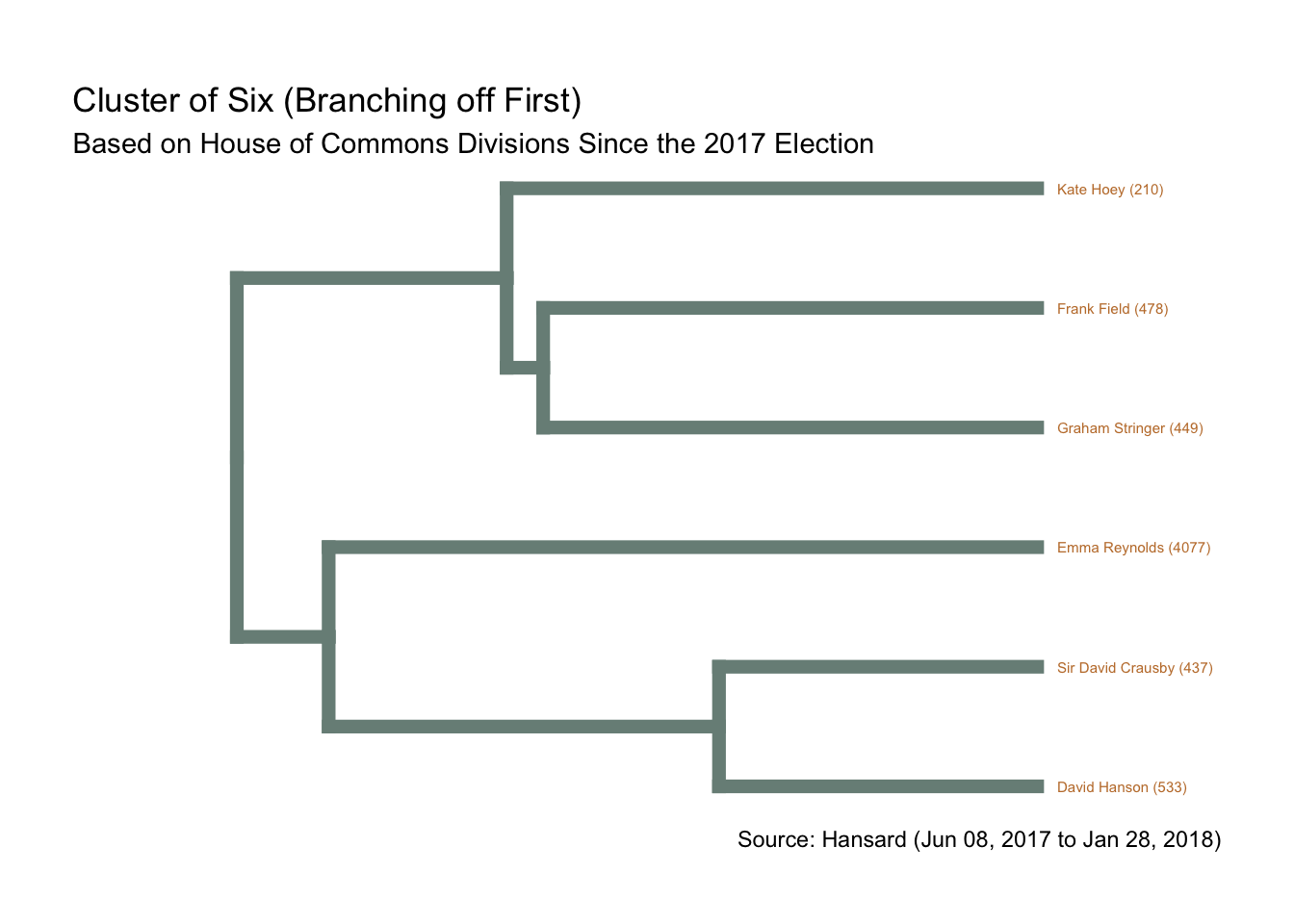Summarising and sorting the total votes by MP tells me that the “cluster of six” MPs are among the eight MPs voting the fewest times. And I can, for example, verify the record for Emma Reynolds directly via Hansard.

``````fewest_votes <- votes |>
left_join(mps, by = join_by(mp == ID)) |>
summarise(n_lobby = n(), .by = c(full_name_value, lobby)) |>
rename(mp = full_name_value) |>
pivot_wider(names_from = "lobby", values_from = "n_lobby") |>
mutate(total = aye + no,
mp = fct_reorder(mp, total)) |>
slice_min(n = 10, order_by = total) |>
pivot_longer(cols = -mp) |>
filter(name != "total")

ggplot(aes(mp, value, fill = name)) +
geom_col() +
geom_label(aes(label = value), position = position_stack()) +
scale_fill_manual(values = cols[c(1, 3)]) +
coord_flip() +
labs(title = "Labour MPs Voting Fewest Times",
y = "Votes", x = NULL, fill = NULL)``````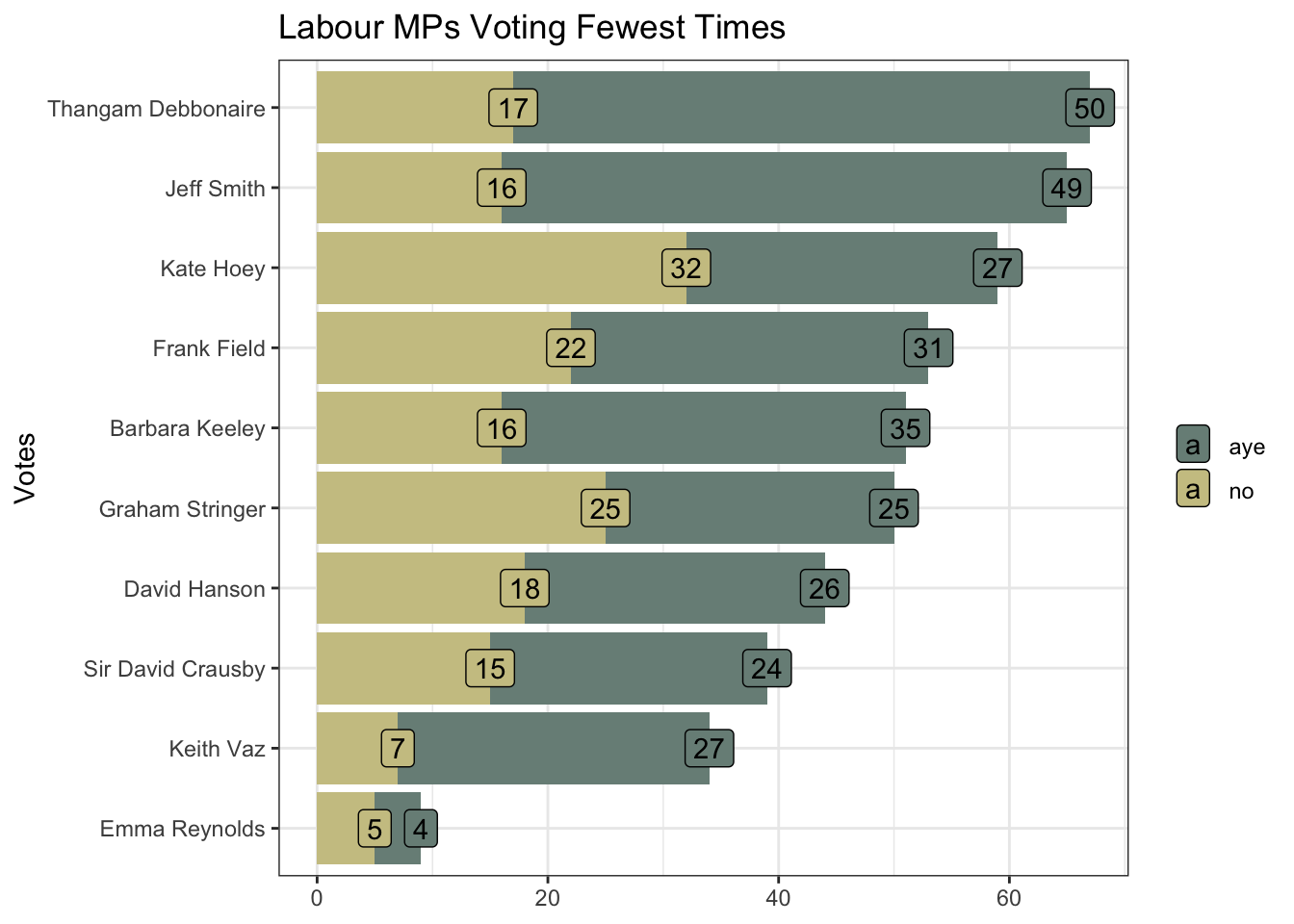Non-voting will not be the only influencing factor. The “distant cluster” will be particularly influenced by a small minority of MPs voting in the opposite direction to the overwhelming majority.

Cook’s Distance visualises these influential outliers. This shows the voting of three MPs, all on the European Union Withdrawal Bill readings, to be particular outliers. All three MPs are in the “cluster of six”.

``````tidy_df <- votes_df |>
pivot_longer(cols = -mp, names_to = "title", values_to = "vote")

mod <- lm(vote ~ ., data = tidy_df)

mod_df <- mod |>
augment() |>
as_tibble()

ggplot(mod_df, aes(title, .cooksd, colour = mp)) +
geom_jitter() +
geom_label_repel(aes(label = if_else(.cooksd > 0.002, mp, NA)), size = 4) +
scale_colour_manual(values = wes_palette(220, name = "Moonrise2", type = "continuous")) +
labs(title = "Cook's Distance") +
coord_flip() +
theme(
panel.border = element_blank(),
axis.text = element_text(size = 6),
legend.position = "none"
)``````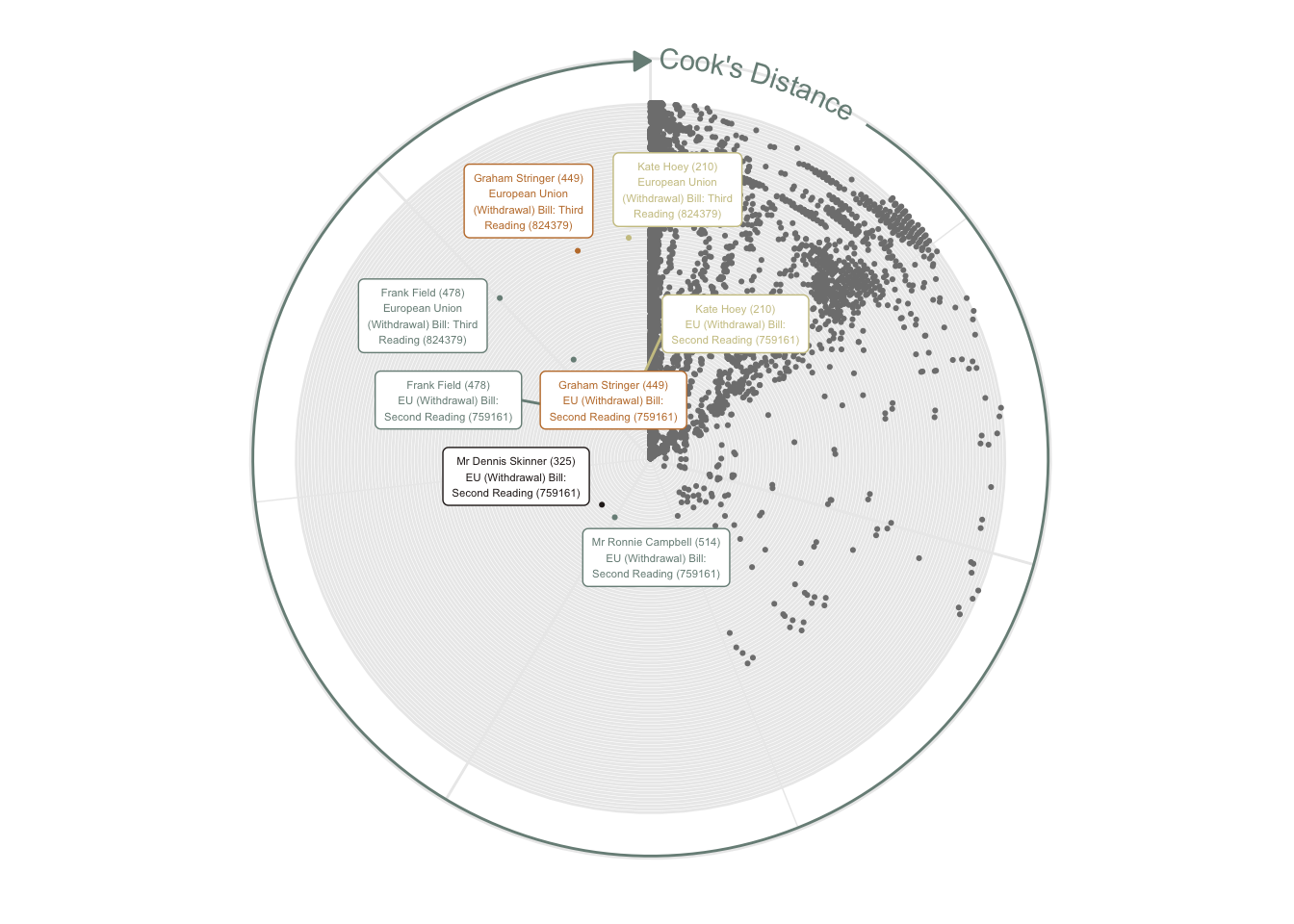``````mod_df |>
filter(str_detect(title, "759161|824379|809989")) |>
mutate(title = str_wrap(title, 30)) |>
ggplot(aes(title, .cooksd, colour = mp)) +
geom_point(size = 4) +
geom_label_repel(aes(label = if_else(.cooksd > 0.0015, mp, NA)), size = 4) +
ggtitle("Cook's Distance") +
theme(
axis.line.x = element_line(color = "grey60"),
axis.text = element_text(size = 8),
legend.position = "none",
axis.title = element_blank()
) +
scale_colour_manual(values = wes_palette(
210, name = "Moonrise2", type = "continuous")) +
coord_flip()``````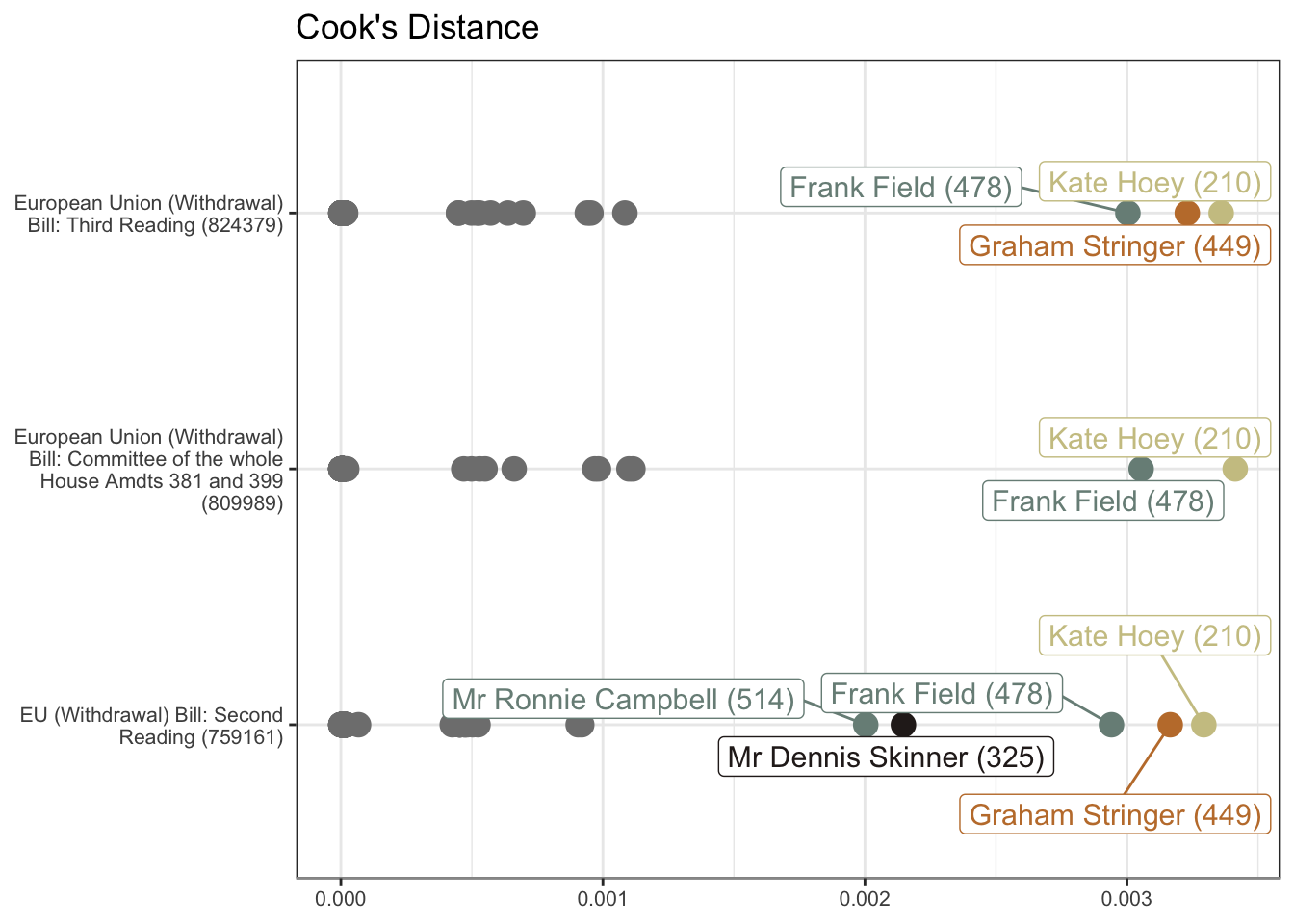## R Toolbox

Summarising below the packages and functions used in this post enables me to separately create a toolbox visualisation summarising the usage of packages and functions across all posts.

``used_here()``
Package Function
base c, cut, labels, library, list, max, min, nrow, readRDS, rev, round, saveRDS
clock date_format, date_parse
conflicted conflict_prefer_all, conflict_scout
corrplot corrplot
dendextend assign_values_to_leaves_nodePar, color_branches, cor.dendlist, set
dplyr across, filter, if_else, join_by, left_join, mutate, n, pick, rename, select, slice_min, summarise, transmute
factoextra fviz_dist, get_clust_tendency
forcats fct_reorder
generics augment
ggplot2 aes, coord_flip, element_blank, element_line, element_text, geom_col, geom_jitter, geom_label, geom_point, geom_text, ggplot, ggtitle, labs, position_stack, scale_colour_manual, scale_fill_manual, theme, theme_bw, theme_set, theme_void, unit
ggrepel geom_label_repel
glue glue
hansard commons_members, mp_vote_record
purrr compact, list_rbind, map, pluck, possibly, reduce
rlang set_names
stats as.dendrogram, cophenetic, cor, dist, hclust, lm, order.dendrogram, reorder
stringr str_c, str_detect, str_replace, str_wrap
tibble as_tibble, tibble
tidyr pivot_longer, pivot_wider
tidyselect ends_with
usedthese used_here
wesanderson wes_palette